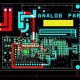# Best Interview Questions and Answer for DC Motor.

In this tutorial, we are going to learn about interview questions of DC motor.

Question1. What is DC motor?

Answer – It c converts electrical energy into mechanical energy.

Question2. What is rule of DC motor operating on the principal?

Answer – It is working of Fleming left hand rule.

Question3. What is the principal of the DC motor?

Answer – It is working on the principal when the current carrying conductor is placed in the magnetic field, mechanical force is experienced on the conductor, and the direction of the force is given as per the Fleming’s left hand rule and hence conductor moves in the direction of force.

F = B I L

F = It is Force

B = It is Magnetic Field Strength W/m2

L = It is Length of the conductor in meter

Question4. What is the second name of D.C. Shunt Motor?

Question5. How to control the speed of DC motor?

Answer – The speed of DC motor can be control by its flux, armature resistance and applied voltage.

Question6. When the armature current of a D.C. motor is maximum?

Answer – When the switched ON that time the armature current of a D.C. motor is maximum.

Question7. What is speed of a D.C. series motor at no load?

Answer – Infinity speed of a D.C. series motor at no load.

Question8. What is starting resistance for D.C. shunt Motor?

Question9. Which type of motor is used in trains and what is the rating of supply used?

Answer – DC series motor is used in trains to get high starting torque while starting of the trains and the operating voltage is 1500V DC.

Question10. How can we change the direction of rotation of DC series motor?

Answer – We can change the direction of rotation of DC series motor by interchanging field terminals.

Question11. What will happen if the field of a DC motor gets opened while motor is running?

Answer – The motor will attain dangerously high speed.

Question12. Why we use starters in DC Motor?

Answer – It is use to restrict armature current as there is no back EMF while starting.

Question13. What will happen if a DC motor is connected across the AC supply?

Answer – If a DC motor is connected across the AC supply then motor will burn due to heat production in field winding by eddy current.

Question14. How many types of DC motor?

Answer – 1. Shunt Motor 2. Series Motor 3. Compound Motor 4. Separately excited motor 5. PMDC motor.

Question15. What is difference between AC & DC motor?

Answer – Dc motor are powered from direct current (DC), such as batteries, DC power supplies and AC motor are powered from alternating current.